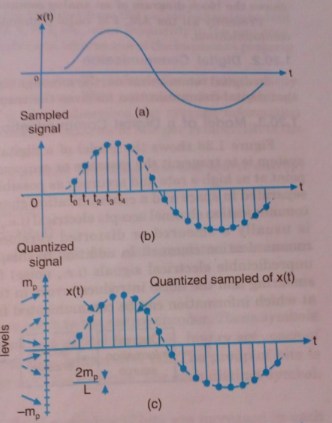# Conversion of Analog Signals to Digital Signals

In communication systems, sometimes it happens that we are available with an analog signal, and we have to transmit a digital signal for that particular application.

In such cases, we have to convert the analog signal to digital signal. That means that we have to convert a continuous time signal in the form of digits.

To see how a signal can be converted from analog signal to digital form, let us consider an analog signal x(t) as shown in fig.1(a).Fig.1 : (a) An Analog Signal, (b) Samples of Analog signal, (c) Quantization

First of all , we get sample of this signal according to the sampling theorem.

For this purpose, we mark the time-instants t0, t,tand so  on , at equal time-intervals along the time axis.

At each of these time-instants , the magnitude of the signal is measured and thus samples of the signal are taken. Fig.1(b) shows a representation of the signal of fig.1(a) in terms of its samples.

Now, we can say that the signal in fig.1(b) is defined only at the sampling instants.

This means that, it no longer is a continuous function of time, but rather, it is a discrete-time signal.

However, since the magnitude of each sample can take any value in a continuous range, the signal in fig.1(b) is still an analog signal.

This difficulty is neatly resolved by a process known as quantization. In quantization, the total amplitude range which the signal may occupy is divided into a number of standard levels.

As shown in fig.1(c), amplitudes of the signal x(t) lie in the range (-m, mp) which is partitioned into L intervals, each of magnitude Δv = 2mp / L .

Now, each sample is approximated  or rounded off to the nearest quantized level as shown in fig.1(c) .

Since each sample is now approximated to one of the L numbers, therefore, the information is digitized.

The quantized signal is an approximation of the original one. We can improve the accuracy of the quantized signal to any desired degree simply by increasing the number of levels L .